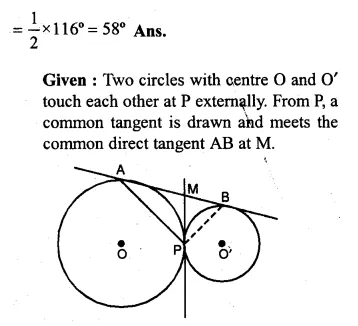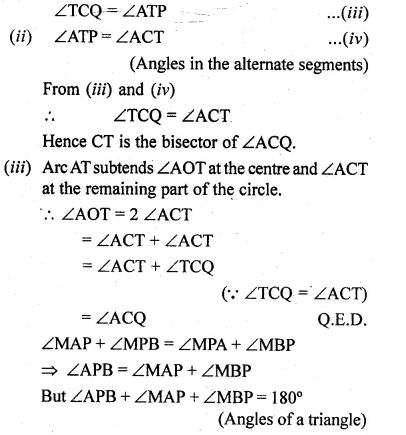Guru

# Ques (b) In the figure (ii) given below, O and O’ are centres of two circles touching each other externally at the point P. The common tangent at P meets a direct common tangent AB at M. Prove that: (i) M bisects AB (ii) ∠APB = 90°.

• 0

One of the most important and exam oriented question from Chapter name- circles
Topic – Angle properties of circles
Chapter number- 15

In this question we have a figure in which it is given that O and O’ are centres of two circles touching each other externally at the point P.

The common tangent at P meets a direct common tangent AB at M.

Now using these facts we have to prove that: (i) M bisects AB (ii) ∠APB = 90°.
ICSE Avichal publication
Understanding ICSE Mathematics
Question 39(b)

Share

1.